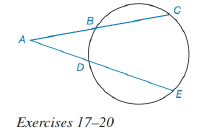Chapter 6.3, Problem 20EElementary Geometry For College St...

7th Edition
Alexander + 2 others
ISBN: 9781337614085

Solutions

Chapter
SectionElementary Geometry For College St...

7th Edition
Alexander + 2 others
ISBN: 9781337614085
Textbook Problem

For Exercises 17 to 2, See Theorem 6.3.6.Given: A B = 5 , B C = 6 , A D = 6 Find: AE.To determine

To find:

The value of AE

Explanation

The diagrammatic representation is given below,

Theorem:

If secant segments are drawn to a circle from an external point, then the products of the length of each secant with the length of its external segment are equal.

By using the theorem 6.3.5 to get the following,

We know that

AC=AB+BCAC=5+6AC=11

Still sussing out bartleby?

Check out a sample textbook solution.

See a sample solution

The Solution to Your Study Problems

Bartleby provides explanations to thousands of textbook problems written by our experts, many with advanced degrees!

Get Started

Expand each expression in Exercises 122. (y+3)(y+4)

Finite Mathematics and Applied Calculus (MindTap Course List)

Show that if x+312, then |4x + 13| 3.

Single Variable Calculus: Early Transcendentals, Volume I

f(x) = 12x x3 has a local maximum at: a) x = 0 b) x = 2 c) x = 2 d) f does not have a local maximum

Study Guide for Stewart's Single Variable Calculus: Early Transcendentals, 8th